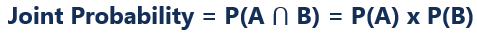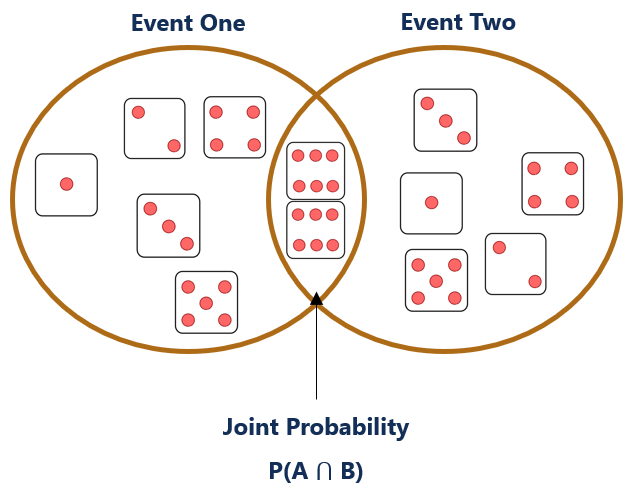# Joint Probability

The probability that two events will both occur

## What is a Joint Probability?

A joint probability, in probability theory, refers to the probability that two events will both occur. In other words, joint probability is the likelihood of two events occurring together.### Formula for Joint ProbabilityWhere:

• P(A ⋂ B) is the notation for the joint probability of event “A” and “B”.
• P(A) is the probability of event “A” occurring.
• P(B) is the probability of event “B” occurring.

### Joint Probability and Independence

For joint probabilities to work, the events must be independent. In other words, the events must not be able to influence each other. To determine whether two events are independent or dependent, it is important to ask whether the outcome of one event would have an impact on the outcome of the other event. If the outcome of one event does not affect the outcome of the other event, the events are independent.

An example of dependent events is the probability of the clouds in the sky and the probability of rain on that day. The probability of clouds in the sky has an impact on the probability of rain that day and are, therefore, dependent events.

An example of independent events is the probability of getting head on two coin tosses. The probability of getting a head on the first coin toss does not have an impact on the probability of getting heads on the second coin toss.

### Visual Representation

A joint probability can be visually represented through a Venn diagram. Consider the joint probability of rolling two 6’s in a fair six-sided dice:Shown on the Venn diagram above, the joint probability is where both circles overlap each other. It is called the “intersection of two events.”

### Examples

The following are examples of joint probability:

#### Example 1

What is the joint probability of rolling the number five twice in a fair six-sided dice?

Event “A” = The probability of rolling a 5 in the first roll is 1/6 = 0.1666.

Event “B” = The probability of rolling a 5 in the second roll is 1/6 = 0.1666.

Therefore, the joint probability of event “A” and “B” is P(1/6) x P(1/6) = 0.02777 = 2.8%.

#### Example 2

What is the joint probability of getting a head followed by a tail in a coin toss?

Event “A” = The probability of getting a head in the first coin toss is 1/2 = 0.5.

Event “B” = The probability of getting a tail in the second coin toss is 1/2 = 0.5.

Therefore, the joint probability of event “A” and “B” is P(1/2) x P(1/2) = 0.25 = 25%.

#### Example 3

What is the joint probability of drawing a number ten card that is black?

Event “A” = The probability of drawing a 10 = 4/52 = 0.0769

Event “B” = The probability of drawing a black card = 26/52 = 0.50

Therefore, the joint probability of event “A” and “B” is P(4/52) x P(26/52) =  0.0385 = 3.9%.

### More Resources

CFI is the official provider of the global Financial Modeling & Valuation Analyst (FMVA)™ certification program, designed to help anyone become a world-class financial analyst. To keep advancing your career, the additional resources below will be useful:

• Basic Statistics Concepts in Finance
• Empirical Probability
• Normal Distribution
• Subjective Probability

### Financial Analyst Certification

Become a certified Financial Modeling and Valuation Analyst (FMVA)® by completing CFI’s online financial modeling classes and training program!